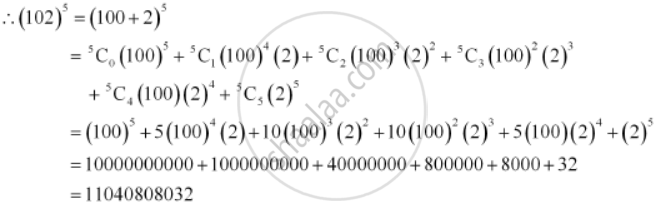Share

# Using Binomial Theorem, Evaluate (102)5 - Mathematics

#### Question

Using Binomial Theorem, evaluate (102)5

#### Solution

102 can be expressed as the sum or difference of two numbers whose powers are easier to calculate and then, Binomial Theorem can be applied.

It can be written that, 102 = 100 + 2Is there an error in this question or solution?